# Statistics & Probability: Fundamentals 2

The second part of our fundamentals. Keep in mind, if you do not already know some of these definitions, write them down on flashcards and learn their definitions and formulas as they were vocabularies.

This time, we take the context for our examples that we already have seen in Fundamentals 1 for some definitions. We simply see the history, say starting from 1945, of the day temperature of a typically warm spring day, say May, 23rd, in Copenhagen.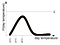History of day temperature in Copenhagen on May, 23rd

Sampling: Randomly choosing a value such that the probability of picking any particular value is given by a probability distribution.
Example: We randomly pick a temperature of all the temperatures ever being recorded in Copenhagen on May, 23rd. This could be18°C. Note that chances to pick a temperature that has occurred more often than others are higher. That’s why chances to pick a temperature closer to the mean of the distribution (circa 20°C) are higher.

We will now introduce a few types of distributions. We don’t refer our example to it, but they are still paramount to remember.

Bernoulli distribution: probability distribution over a two-valued (= binary = true or false = 1 or 0) random variable. The Bernoulli distribution has one parameter p which is the probability of the value true and is written as Bernoulli(p).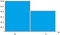Bernoulli distribution for p = 0.4

Categorical distribution: probability distribution for a set of discrete random variables. You can see it as the generalisation of the Bernoulli distribution. There, you only have two possible values, but in a categorical you can have more. We could use it to determine the probabilities of an input belonging to a certain class or weight as in the following graph shown.

Uniform distribution: probability distribution where every possible value is equally probable. It is always defined with boundaries a and b, and the probability is given by 1/(b-a), because the sum of all probabilities must be 1. In other words, the “space under the line” must be 1.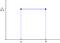Uniform distribution with boundaries a and b

Gaussian distribution: the “bell-shape” distribution very often used for real-valued random variables (also called normal distribution).

Joint distribution: a probability distribution over multiple variables which gives the probability of the variables jointly taking a particular configuration of values. For example, p(X,Y) is a joint distribution over the random variables X and Y.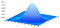Joint distribution of two Gaussian distributed random variables X and Y.

Conditional probability: a probability of one random variable given a another random variable has a particular value. Formally, we say “the conditional probability of X given Y is the probability of event X when event
Y is known”.

It might be not too easy to grasp in the beginning, so let’s look at a probability tree for an intuitive explanation.
Example: We have the temperature distribution of May, 23rd, our T1, but do not know it for May, 24th, our T2. We calculate the conditional probability when we, taken the left branch in the following graph into account, ask ourselves “how warm will it be tomorrow when it was today only 4°C?”. The chance that it is on T2 25°C are with 0.2 pretty low, whereas the chances that it’ll be 9°C are with 0.8 higher. You can do the same for the right branch. Chances that it’ll be 20°C on the 24th after it has been 22°C on the 23rd are with 0.6 higher than 15°C with 0.4.

Chain rule of probability: it’s nothing more than rewriting what we already know from the conditional probability. It’s the product of the distribution over Y and the distribution over X conditioned on the value of Y. In other words, it’s the probability that both events X and Y occur at once.

Bayes’ theorem: the most important, but not very complicated rule for the rest of your journey. p(X|Y) is the posterior probability of X given data Y, p(Y|X) is the likelihood or model evidence of data fitting to your model configurations, p(X) is the belief in form of a prior probability, and p(Y) is the data distribution.

Julia Galef’s explanation or Arbital’s tutorial are superb and might help you understanding its intuitions. Watch the video a few times and do the tutorial, you should see the world through Bayesian eyes.

Sum rule of probability: the probability distribution over a random variable X is obtained by summing the joint distribution p(X,Y) over all values of Y.

Marginal distribution: the distribution over a random variable computed by using the sum rule to sum a joint distribution over all other variables in the distribution. The process of summing a joint distribution to compute a marginal distribution is called marginalisation. Given two random variables X and Y whose joint distribution p(X, Y) is known, the marginal distribution of X is simply the probability distribution of X averaging over all possible values of Y. It is the probability distribution of X when the value of Y is unknown. This is typically calculated by summing (Y is discrete) or integrating (Y is continuous) the joint probability distribution over Y.

Independence: random variables are independent if knowing about X tells us nothing about Y. They are independent if and only if

Conditional independence: a random variable X is conditionally independent from Y given Z, what we write in the following manner:

See it as a graphical model, and it’s totally intuitive. As soon as we know something about Z, we don’t need any information about Y to know something about X, and we don’t need any information about X to know something about Y.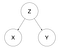Z is known.

Independent and identically distributed: two or more data samples can independent when drawing from one data sample does not influence any following draw, and identically distributed when their means and variances are the same. It’s abbreviated as i.i.d. Since we can never be totally sure that two or more samples are totally independent and identically distributed, you’re playing safe when you say “we assume samples are i.i.d”.

Example: We draw two temperatures of our data set, say 22°C and 15°C. These temperatures display the temperature of two May, 23rd’s in the history of Copenhagen. Do you think the draws are independent? We cannot say it for sure, but taken into account that there were about 365 days between them, they probably are independent. In contrast, we can say with growing sample sizes, i.e. drawing more temperatures at once from the total history, we can confidentially say that the samples are identically distributed, because they originate from the same distribution.

likelihood function: a measure how well the data summarizes the parameters of our model, i.e. our probability distribution.
Later when we’ll have progressed in probabilistic models, we will encounter the log likelihood in a so-called cost function that is commonly the maximum log likelihood.

Inference: the process of computing probability distributions over certain specified random variables, usually after observing the value of some other variables in the model.

Covariance matrix: a measure of the joint variability of two random variables.

Correlation matrix: correlation is a special case of covariance which can be obtained when the data is standardized. Standardized means each observation is subtracted by the mean and divided by the standard deviation. Therefore, it ranges from -1 to 1, i.e. its value can directly be interpreted as “good” or “bad”. So, do normalize your data before you compute the correlation between two random variables.

Causation: causation exists between two random variables, if changes in one is the reason of changes in the other. An edge between two random variables implies this, formally called a causal relation. It’s a crucial, but often overlooked idea, and you might have been reminded by the idiom “Correlation Does Not Imply Causation”.

Example: imagine it’s May, 23rd today, around 22°C, and you’re walking around Copenhagen. You’ll see many pedestrians eating ice-cream and ask yourself whether that’s because it’s quite hot today or do Danes just like ice-cream very much. After having spent a few more days in Copenhagen with temperatures ranging from 10°C to 25°C, you can guess that it’s probably because of the high temperature. You have just drawn a causal relation between the random variables “temperature” and “eating ice-cream”. But, you could also observe that pedestrians go shopping a lot the warm days you spend in Copenhagen, seemingly more than on the colder days. Does that also imply a causal relation? You cannot be certain, because there could simply has been public holidays on the cold days and most stores were closed, so less people were attracted to go shopping.

Written by

## Felix Laumann

#### helping you with the first steps into Bayesian deep learning | PhD Student at Imperial College London | Research Scientist at NeuralSpace

Welcome to a place where words matter. On Medium, smart voices and original ideas take center stage - with no ads in sight. Watch
Follow all the topics you care about, and we’ll deliver the best stories for you to your homepage and inbox. Explore
Get unlimited access to the best stories on Medium — and support writers while you’re at it. Just \$5/month. Upgrade